• Opencv图像处理基本操作 1基本数据类型 图像有若干个通道，灰度图像只有一个通道，而彩色具有红，绿，蓝组成，但是OpenCv以逆序的方式来存储三个分量，还可以使用第四个透明度(alpha)，可以使用img.channels()获取...
Opencv图像处理基本操作
1基本数据类型

图像有若干个通道，灰度图像只有一个通道，而彩色具有红，绿，蓝组成，但是OpenCv以逆序的方式来存储三个分量，还可以使用第四个透明度(alpha)，可以使用img.channels()获取图像通道个数。

使用若干个位存储一副图像的每个像素，这被称为图像的深度，灰度图像为8位，即0-255个灰度级，可以用img.depth()获得图像的深度，其返回值为：

CV_8U - 8-bit unsigned integers ( 0..255 )  CV_8S - 8-bit signed integers ( -128..127 )  CV_16U - 16-bit unsigned integers ( 0..65535 )  CV_16S - 16-bit signed integers ( -32768..32767 )  CV_32S - 32-bit signed integers ( -2147483648..2147483647 )  CV_32F - 32-bit floating-point numbers ( -FLT_MAX..FLT_MAX, INF, NAN )  CV_64F - 64-bit floating-point numbers ( -DBL_MAX..DBL_MAX, INF, NAN )

对于灰度图像和彩色图像，最常见的是CV_8U.
Mat img=imread("lena.png",IMREAD_GRAYSCALE);
Mat fp;
img.convertTo(fp,CV_32F);//改变图像的深度

123
2 像素级访问
1. 第一种方法：模板函数at<>
uchar pixel=img.at<uchar>(0,0);  //获得灰度图像0，0点像素
Vec3b pixel=img.at<Vec3B>(0,0);  //获得3波段图像的第一个波段（0，0）像素。

12
第一种方法，效率不高，必须定位到他所在的位置
2. 第二种方法：函数ptr
他返回图像特定行的指针。因此可以得到每一行的数据，时间复杂度降低，  如下代码获取一副彩色图像的每个像素值。
//时间复杂度大大降低！！！
uchar R,G,B;
for (int i=0;i<img.rows;i++)    //遍历行
Vec3b pixRow=img.ptr<Vec3b>(i);
for (int j=0;j<img.cols;j++) {   //遍历**列**
B=pixRow[j];
G=pixRow[j];
R=pixRow[j];
}

123456789
测量程序用时可用函数：

double to=(double)getTickCount();  elapsed=((double)getTickCount()-to)/getTickFrenquency()

图像位运算
可以用掩码对一个图像进行处理，位元算有：

void bitwise_and(InputArray src1, InputArray src2, OutputArray dst, InputArray mask=noArray())  其中src1是原始的图像，src2是掩码，dst为输出

一个例子：
#include<opencv2\opencv.hpp>
#include <iostream>
using namespace cv;
using namespace std;
int main() {
if (img.empty())
cout << "cannot load image" << endl;
imshow("Origin", img);
Mat mask(img.rows, img.cols,CV_8UC3, Scalar(0, 0,0 ));
circle(mask, Point(img.rows / 2, img.cols / 2-35), 220,Scalar(255,255,255),-1);  //画一个圆
//执行位操作
Mat r;
imshow("Bit_and", r);
waitKey(0);
return 0;
}

12345678910111213141516171819
如下所示的图像处理过程：  分别为原始图像，掩模，计算后的图像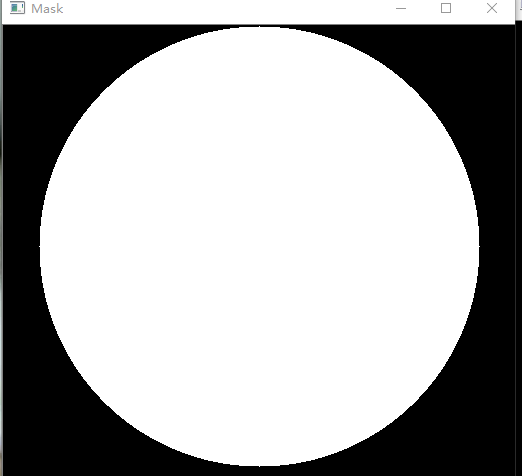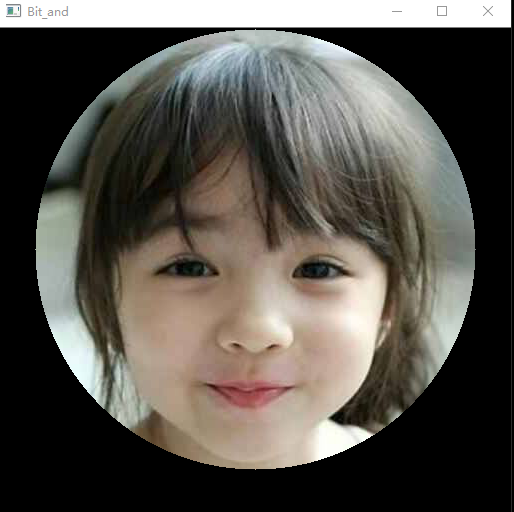1. Adding (blending) two images using OpenCV
将两张图像以线性组合的方式合并成一张图像，注意的是，两张图像的大小应该相同。  g(x) = (1 -a)*f0(x) + a*f1(x)g(x)为生成的矩阵，f0(x),f1(x)为要合并的两个矩阵。a为尺度。  用到的函数原型：  C++:  void addWeighted(InputArray src1, double alpha, InputArray src2, double beta, double gamma, OutputArray dst, int dtype=-1)
#include <opencv2/core.hpp>
#include <opencv2/imgcodecs.hpp>
#include <opencv2/highgui.hpp>
#include <iostream>
#include <string>
using namespace cv;
using namespace std;
int main(int argc, char** argv)
{
double alpha = 0.5; double beta; double input;
Mat src1, src2, dst;
/// Ask the user enter alpha
std::cout << " Simple Linear Blender " << std::endl;
std::cout << "-----------------------" << std::endl;
std::cout << "* Enter alpha [0-1]: ";
std::cin >> input;
/// We use the alpha provided by the user if it is between 0 and 1
if (input >= 0.0 && input <= 1.0)
alpha = input;
/// Read image ( same size, same type )
/// Create Windows
namedWindow("Linear Blend", 1);
beta = (1.0 - alpha);
addWeighted(src1, alpha, src2, beta, 0.0, dst);
imshow("Linear Blend", dst);
waitKey(0);
return 0;
}

1234567891011121314151617181920212223242526272829303132
当a=0时,此时只有window的logo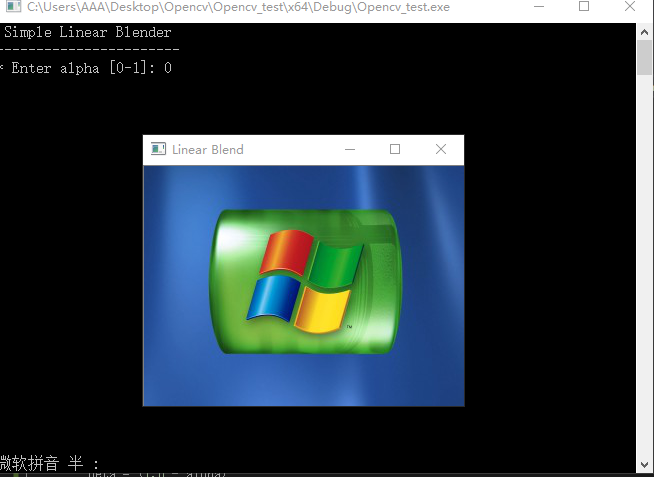a=0.5时如图所示：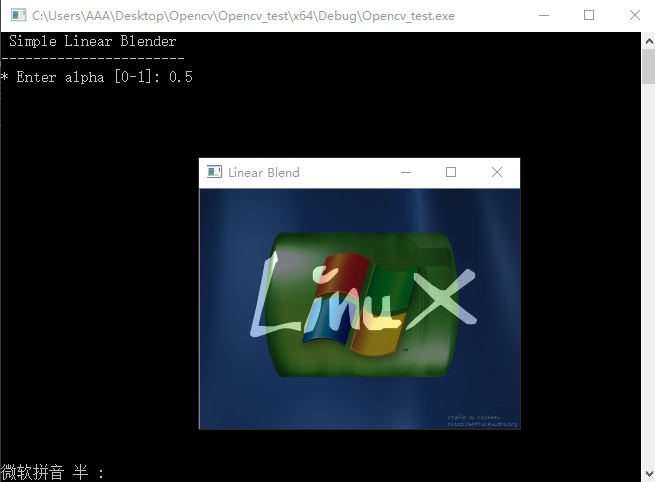2. Changing the contrast and brightness of an image
改变图像的对比度和亮度  基础的公式为：  g(i; j) = a*f(i,j) + b  where i and j indicates that the pixel is located in the i-th row and j-th column.  获得一个图片的像素我们用image.at<Vec3b>(y,x)[c] 这里的y为行，x为列，c代表R, G or B (0, 1 or 2)
int main(int argc, char** argv)
{
double alpha; /**< Simple contrast control */
int beta; /**< Simple brightness control */
/// Read image given by user
Mat new_image = Mat::zeros(image.size(), image.type());  //copy the origin picture size,and type
/// Initialize values
std::cout << " Basic Linear Transforms " << std::endl;
std::cout << "-------------------------" << std::endl;
std::cout << "* Enter the alpha value [1.0-3.0]: "; std::cin >> alpha;
std::cout << "* Enter the beta value [0-100]: "; std::cin >> beta;
/// Do the operation new_image(i,j) = alpha*image(i,j) + beta
for (int y = 0; y < image.rows; y++){
for (int x = 0; x < image.cols; x++){
for (int c = 0; c < 3; c++){
new_image.at<Vec3b>(y, x)[c] =
saturate_cast<uchar>(alpha*(image.at<Vec3b>(y, x)[c]) + beta);//saturate_cast to make sure the values are valid.
}
}
}
namedWindow("Original Image", 1);
namedWindow("New Image", 1);
imshow("Original Image", image);
imshow("New Image", new_image);
waitKey();
return 0;
}

12345678910111213141516171819202122232425262728
示例如下：可以看到改变的图片的对比度和亮度。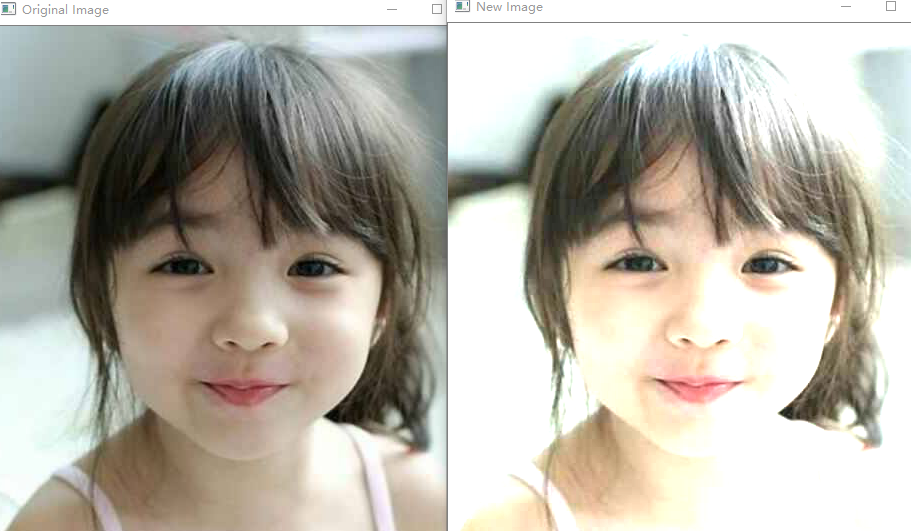这里可以用函数image.convertTo(new_image, -1, alpha, beta);来代替for循环，它会更有效率。
Basic Drawing
1. 定义一个点 2D
Point pt;
pt.x = 10;
pt.y = 8;
Point pt = Point(10, 8);

1234
2. 画椭圆ellipse原型  void ellipse(InputOutputArray img, Point center, Size axes,  double angle, double startAngle, double endAngle,  const Scalar& color, int thickness = 1,  int lineType = LINE_8, int shift = 0);
后面三个为默认的参数，可以不写。
//自己写的函数，指定img，和角度
void MyEllipse(Mat img, double angle)
{
int thickness = 2;
int lineType = 8;
ellipse(img,
Point(w / 2, w / 2),
Size(w / 4, w / 16),
angle,
0,
360,
Scalar(255, 0, 0),  //为颜色
thickness,
lineType);
}
//**调用方法：**
MyLine(rook_image, Point(0, 15 * w / 16), Point(w, 15 * w / 16));

1234567891011121314151617
3. 画线段
函数原型  void line(InputOutputArray img, Point pt1, Point pt2, const Scalar& color,  int thickness = 1, int lineType = LINE_8, int shift = 0);
Random generator and text with OpenCV
随机数产生类Random Number generator class (RNG)
RNG rng( 0xFFFFFFFF );//创建一个RNG类，并对其进行初始化
//在[a,b)中随机产生一个数
C++: int RNG::uniform(int a, int b)
C++: float RNG::uniform(float a, float b)
C++: double RNG::uniform(double a, double b)
//a – lower inclusive boundary of the returned random numbers.
//b – upper non-inclusive boundary of the returned random numbers.
RNG rng;
// always produces 0
double a = rng.uniform(0, 1);
// produces double from [0, 1)
double a1 = rng.uniform((double)0, (double)1);
// produces float from [0, 1)
double b = rng.uniform(0.f, 1.f);
// produces double from [0, 1)
double c = rng.uniform(0., 1.);
// may cause compiler error because of ambiguity:
//  RNG::uniform(0, (int)0.999999)? or RNG::uniform((double)0, 0.99999)?
double d = rng.uniform(0, 0.999999);

123456789101112131415161718192021222324
Random color
一副彩色图像由R,G,B组成，可用RNG产生随机的颜色。
static Scalar randomColor( RNG& rng )
{
int icolor = (unsigned) rng;
return Scalar( icolor&255, (icolor>>8)&255, (icolor>>16)&255 );
}

12345
Put out the text
获得一个字符串的宽度和高度：  C++: Size getTextSize(const string& text, int fontFace, double fontScale, int thickness, int* baseLine)  例如：  Size textsize = getTextSize("OpenCV forever!", CV_FONT_HERSHEY_COMPLEX, 3, 5, 0);  将其输出:  C++: void putText(Mat& img, const string& text, Point org, int fontFace, double fontScale, Scalar color, int thickness=1, int lineType=8, bool bottomLeftOrigin=false )
转载自：https://blog.csdn.net/taoyanqi8932/article/details/52205109

展开全文• 只能显示彩色图像，灰度图像显示有问题，经查找，是没有设置图像调色板的原因 图片控件宽度不为4的倍数 显示错误， 修改 代码 /***************************************************** * * 函数...
VS2015+opencv3.0 MFC显示图片中方法三在使用时，
只能显示彩色图像，灰度图像显示有问题，经查找，是没有设置图像调色板的原因图片控件宽度不为4的倍数 显示错误， 修改
代码
 
/*****************************************************  *  * 函数名称： void DrawcvMat(cv::Mat m_cvImg, UINT ID)  * 函数功能： 在picture control中显示图像，设置Freame或者rectangle都可以  *  * ****************************************************/  void CMFC_showImageDemoDlg::DrawcvMat(cv::Mat m_cvImg, UINT ID)  {   cv::Mat img;   CRect rect;    GetDlgItem(ID)->GetClientRect(&rect);  if (rect.Width()%4 != 0)  {  rect.SetRect(rect.left, rect.top, rect.left + (rect.Width() + 3) / 4 * 4, rect.bottom); //调整图像宽度为4的倍数  GetDlgItem(ID)->SetWindowPos(NULL, rect.left, rect.top, rect.Width(), rect.Height(), SWP_NOMOVE);  }   cv::Rect dst(rect.left, rect.top, rect.right, rect.bottom);   cv::resize(m_cvImg, img, cv::Size(rect.Width(), rect.Height())); //使图像适应控件大小    unsigned int m_buffer[sizeof(BITMAPINFOHEADER) + sizeof(RGBQUAD)*256];   BITMAPINFO* m_bmi = (BITMAPINFO*) m_buffer;   BITMAPINFOHEADER* m_bmih = &(m_bmi->bmiHeader);   memset(m_bmih, 0, sizeof(*m_bmih));   m_bmih->biSize = sizeof(BITMAPINFOHEADER);   m_bmih->biWidth = img.cols; //必须为4的倍数  m_bmih->biHeight = -img.rows; //在自下而上的位图中 高度为负  m_bmih->biPlanes = 1;   m_bmih->biCompression = BI_RGB;   m_bmih->biBitCount = 8 * img.channels();    if (img.channels() == 1) //当图像为灰度图像时需要设置调色板颜色  {  for (int i = 0; i < 256; i++)  {  m_bmi->bmiColors[i].rgbBlue = i;  m_bmi->bmiColors[i].rgbGreen = i;  m_bmi->bmiColors[i].rgbRed = i;  m_bmi->bmiColors[i].rgbReserved = 0;  }  }   CDC *pDC = GetDlgItem(ID)->GetDC();   ::StretchDIBits( pDC->GetSafeHdc(), 0, 0, rect.Width(), rect.Height(), 0, 0, rect.Width(), rect.Height(), img.data, (BITMAPINFO*) m_bmi, DIB_RGB_COLORS, SRCCOPY );   ReleaseDC(pDC);   }
来张效果图 (虽然lenna是经典，但我还是更喜欢胖迪^-^)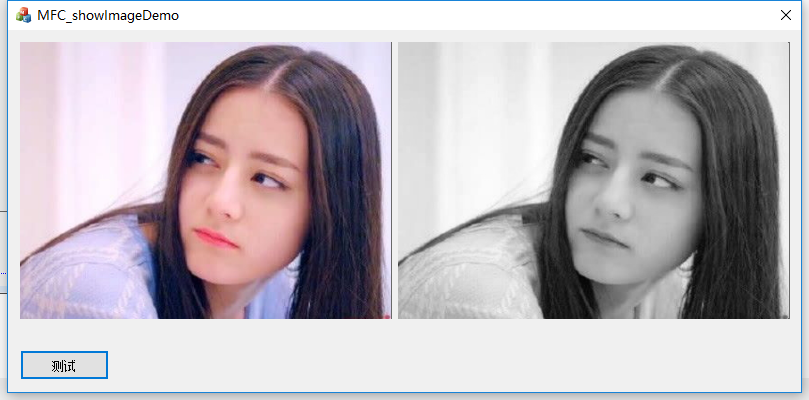展开全文• 项目需求中需要把两个图片横向拼接到一起，最初的想法是遍历每个Mat的每个元素值，拷贝到新的Mat中，但是测试发现：性能问题堪忧啊，两个1920x1080的图片拼接就要耗时500多毫秒。所以就要另辟蹊径了~ 正文 这里...
序
项目需求中需要把两个图片横向拼接到一起，最初的想法是遍历每个Mat的每个元素值，拷贝到新的Mat中，但是测试发现：性能问题堪忧啊，两个1920x1080的图片拼接就要耗时500多毫秒。所以就要另辟蹊径了~
正文
这个拼接函数可以实现两个相同类型（如：同为单通道灰度图、或者3通道彩色图、或4通道argb图）、尺寸不同的两个图片（Mat）的水平方向的拼接。
这里使用了Mat类的 colRange() 和 copyTo().
colRange方法是指定一个区域范围作为选区，返回这个选区，它也是Mat类型。
copyTo方法就一目了然了，不多介绍。
下面是我测试的代码：
package com.blc.face;

import org.opencv.core.Mat;
import org.opencv.core.Range;

public class Test {

/**
* 横向拼接两个图像的数据（Mat），该两个图像的类型必须是相同的类型，如：均为CvType.CV_8UC3类型
* @author bailichun
* @since 2020.02.20 15:00
* @param m1 要合并的图像1（左图）
* @param m2 要合并的图像2（右图）
* @return 拼接好的Mat图像数据集。其高度等于两个图像中高度较大者的高度；其宽度等于两个图像的宽度之和。类型与两个输入图像相同。
* @throws Exception 当两个图像数据的类型不同时，抛出异常
*/
public Mat concat(Mat m1, Mat m2) throws Exception{

System.out.println("图1 width="+m1.size().width);
System.out.println("图1 height="+m1.size().height);
System.out.println("图2 width="+m2.size().width);
System.out.println("图2 height="+m2.size().height);

if(m1.type() != m2.type()){
throw new Exception("concat:两个图像数据的类型不同！");
}
long time = System.currentTimeMillis();
//宽度为两图的宽度之和
double w = m1.size().width + m2.size().width;
//高度取两个矩阵中的较大者的高度
double h = m1.size().height > m2.size().height ? m1.size().height : m2.size().height;
//创建一个大矩阵对象
Mat des = Mat.zeros((int)h, (int)w, m1.type());

//在最终的大图上标记一块区域，用于存放复制图1（左图）的数据，大小为从第0列到m1.cols()列
Mat rectForM1 = des.colRange(new Range(0, m1.cols()));

//标记出位于rectForM1的垂直方向上中间位置的区域，高度为图1的高度，此时该区域的大小已经和图1的大小相同。（用于存放复制图1（左图）的数据）
int rowOffset1 = (int)(rectForM1.size().height-m1.rows())/2;
rectForM1 = rectForM1.rowRange(rowOffset1, rowOffset1 + m1.rows());

//在最终的大图上标记一块区域，用于存放复制图2（右图）的数据
Mat rectForM2 = des.colRange(new Range(m1.cols(), des.cols()));

//标记出位于rectForM2的垂直方向上中间位置的区域，高度为图2的高度，此时该区域的大小已经和图2的大小相同。（用于存放复制图2（右图）的数据）
int rowOffset2 = (int)(rectForM2.size().height-m2.rows())/2;
rectForM2 = rectForM2.rowRange(rowOffset2, rowOffset2 + m2.rows());

//将图1拷贝到des的指定区域 rectForM1
m1.copyTo(rectForM1);
//将图2拷贝到des的指定区域 rectForM2
m2.copyTo(rectForM2);

System.out.println("图片合并耗时："+(System.currentTimeMillis()-time)+"ms");
return des;
}
}


效果图1（两个大小相同的图片拼接）：效果图2（两个宽度相同，高度不同的图片拼接）：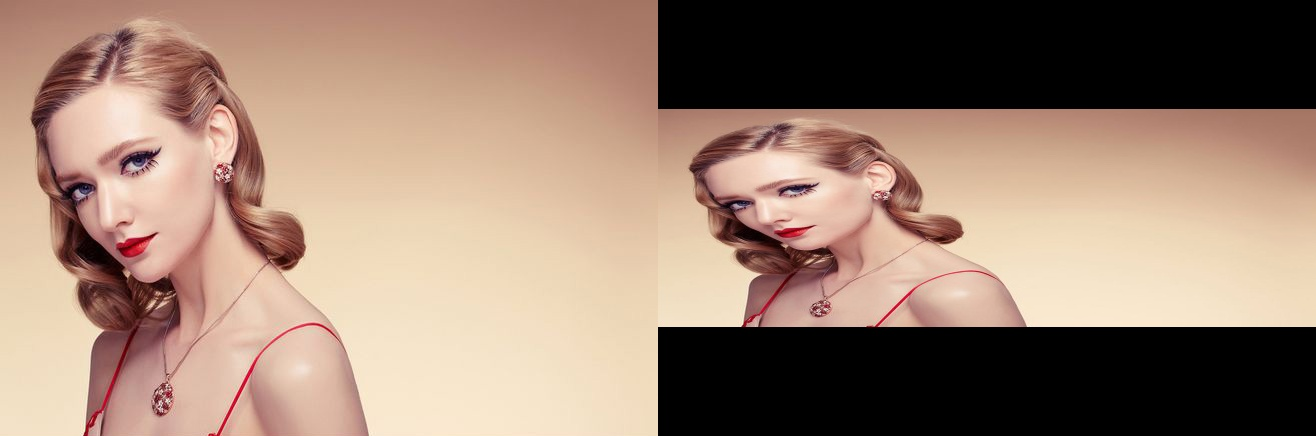效果图3（两个高度相同，宽度不同的图片拼接）：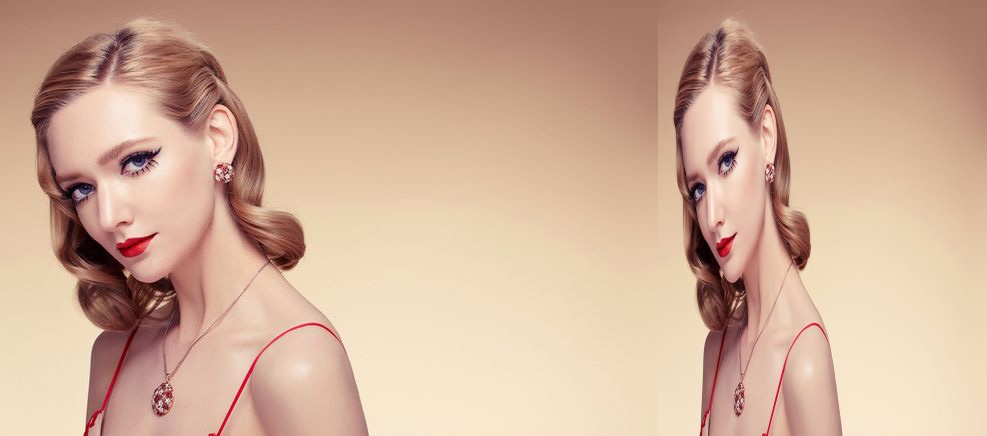这是控制台打印的数据：
图1 width=1920.0
图1 height=1080.0
图2 width=1920.0
图2 height=1080.0
图片合并耗时：8ms

展开全文opencv
• Mat 类早期的 OpenCV 中，使用 IplImage 和 CvMat 数据结构来表示图像。 IplImage和 CvMat 都是 C 语言的结构。 使用这两个结构的问题是内存需要手动管理，开发者必须清楚的知道何时需要申请内存，何时需要释放内存...
Mat 类
早期的 OpenCV 中，使用 IplImage 和 CvMat 数据结构来表示图像。 IplImage和 CvMat 都是 C 语言的结构。 使用这两个结构的问题是内存需要手动管理，开发者必须清楚的知道何时需要申请内存，何时需要释放内存。这个开发者带来了一定的负担，开发者应该将更多精力用于算法设计，因此在新版本的 OpenCV 中引入了 Mat 类。新加入的 Mat 类能够自动管理内存。使用 Mat 类，你不再需要花费大量精力在内存管理上。而且你的代码会变得很简洁，代码行数会变少。但 C++接口唯一的不足是当前一些嵌入式开发系统可能只支持 C 语言，如果你的开发平台支持C++，完全没有必要再用 IplImage 和 CvMat。在新版本的 OpenCV 中，开发者依然可以使用 IplImage 和 CvMat，但是一些新增加的函数只提供了 Mat 接口。
一、Mat 类的定义
如下所示，关键的属性如下方代码所示：  自己也可以在opencv的程序里打开看个究竟。
class CV_EXPORTS Mat
{
public:
//一系列函数
...
/* flag 参数中包含许多关于矩阵的信息，如：
-Mat 的标识
-数据是否连续
-深度
-通道数目
*/
int flags;
//矩阵的维数，取值应该大于或等于 2
int dims;
//矩阵的行数和列数，如果矩阵超过 2 维，这两个变量的值都为-1
int rows, cols;
//指向数据的指针
uchar* data;
//指向引用计数的指针
//如果数据是由用户分配的，则为 NULL
int* refcount;24
//其他成员变量和成员函数
...
};
二.创建 Mat 对象
Mat 是一个非常优秀的图像类，它同时也是一个通用的矩阵类，可以用来创建和操作多维矩阵。 有多种方法创建一个 Mat 对象。  1 构造函数方法  Mat 类提供了一系列构造函数，可以方便的根据需要创建 Mat 对象。 下面是一个使用构造函数创建对象的例子。
Mat M(3,2, CV_8UC3, Scalar(0,0,255));
cout << "M = " << endl << " " << M << endl;
第一行代码创建一个行数（高度）为 3，列数（宽度）为 2 的图像，图像元素是 8 位无符号整数类型，且有三个通道。图像的所有像素值被初始化为(0, 0,255)。由于 OpenCV 中默认的颜色顺序为 BGR，因此这是一个全红色的图像。  关于常见颜色的RGB组合及解释，见下图：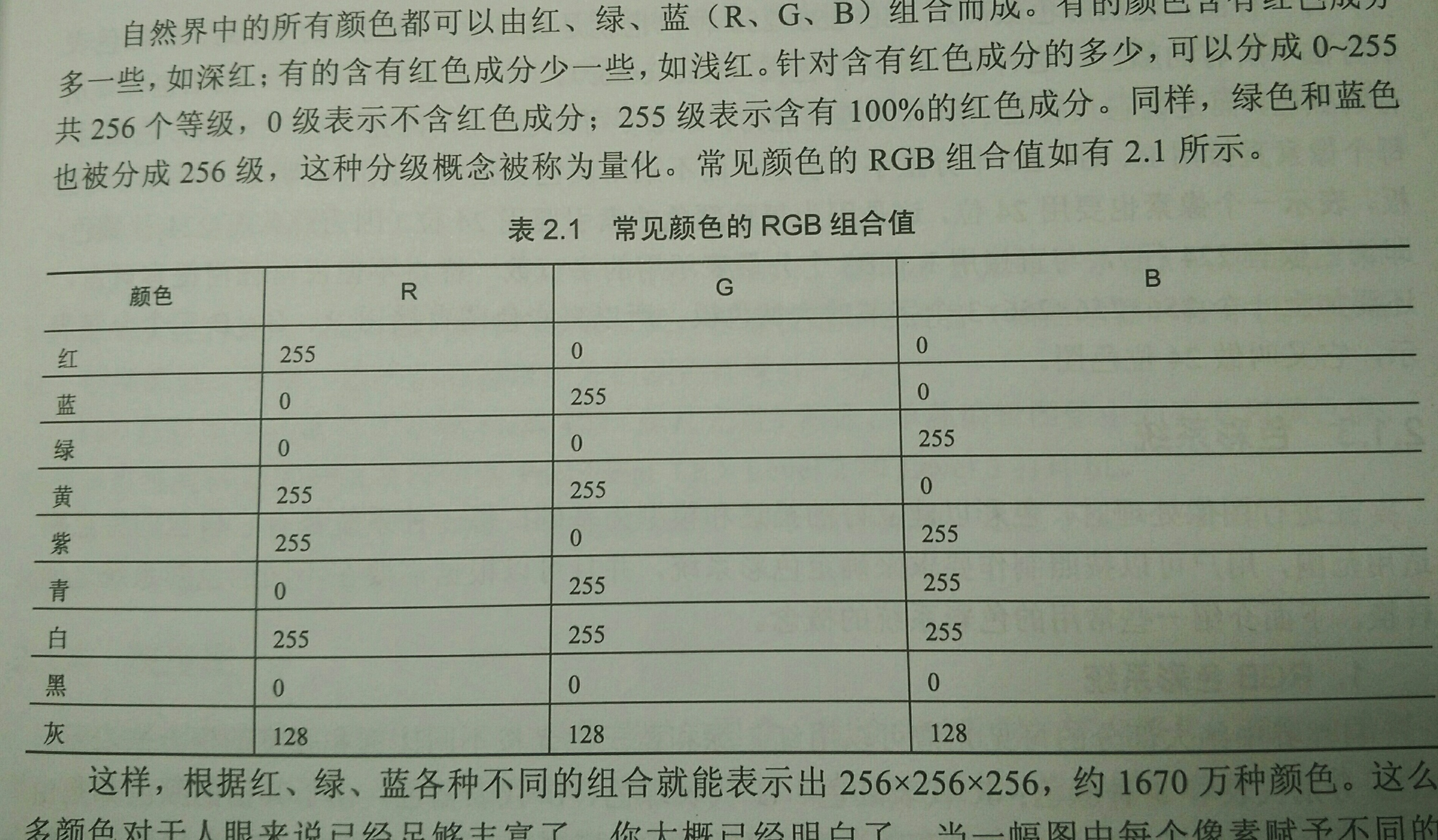第二行代码是输出 Mat 类的实例 M 的所有像素值。Mat 重定义了<<操作符，使用这个操作符，可以方便地输出所有像素值，而不需要使用 for 循环逐个像素输出。  该段代码的输出如下图所示：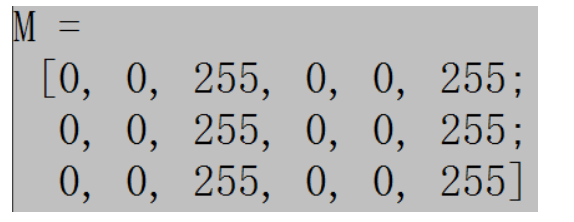常用的构造函数有：  Mat::Mat()  无参数构造方法；
Mat::Mat(int rows, int cols, int type)  创建行数为 rows，列数为 col，类型为 type 的图像；
Mat::Mat(Size size, int type)  创建大小为 size，类型为 type 的图像；
Mat::Mat(int rows, int cols, int type, const Scalar& s)25  创建行数为 rows，列数为 col，类型为 type 的图像，并将所有元素初始化为值 s；
Mat::Mat(Size size, int type, const Scalar& s)  创建大小为 size，类型为 type 的图像，并将所有元素初始化为值 s；
Mat::Mat(const Mat& m)  将 m 赋值给新创建的对象，此处不会对图像数据进行复制， m 和新对象  共用图像数据；
Mat::Mat(int rows, int cols, int type, void* data, size_t step=AUTO_STEP)  创建行数为 rows，列数为 col，类型为 type 的图像，此构造函数不创建图像数据所需内存，而是直接使用 data 所指内存，图像的行步长由 step  指定。
Mat::Mat(Size size, int type, void* data, size_t step=AUTO_STEP)  创建大小为 size，类型为 type 的图像，此构造函数不创建图像数据所需内存，而是直接使用 data 所指内存，图像的行步长由 step 指定。
Mat::Mat(const Mat& m, const Range& rowRange, const Range& colRange)  创建的新图像为 m 的一部分，具体的范围由 rowRange 和colRange 指定，此构造函数也不进行图像数据的复制操作，新图像与 m 共用图像数据；
Mat::Mat(const Mat& m, const Rect& roi)  创建的新图像为 m 的一部分，具体的范围 roi 指定，此构造函数也不进行图像数据的复制操作，新图像与 m 共用图像数据。  这些构造函数中，很多都涉及到类型 type。  type可以是 CV_8UC1，CV_16SC1，…，CV_64FC4 等。里面的  8U 表示 8 位无符号整数， 即unsigned  16S 表示 16 位有符号整数， 即signed  64F表示 64 位浮点数（即 float 类型）
C 后面的数表示通道数，  例如 C1 表示一个通道的图像， C4 表示 4 个通道的图像，以此类推。如果你需要更多的通道数，需要用宏 CV_8UC(n)，例如：  Mat M(3,2, CV_8UC(5));//创建行数为 3，列数为 2，通道数为 5 的图像。
2、 create()函数创建对象  除了在构造函数中可以创建图像，也可以使用 Mat 类的 create()函数创建图像。如果 create()函数指定的参数与图像之前的参数相同，则不进行实质的内存申请操作；如果参数不同，则减少原始数据内存的索引，并重新申请内存。  使用方法如下面例程所示：
Mat M(2,2, CV_8UC3);//构造函数创建图像
M.create(3,2, CV_8UC2);//释放内存重新创建图像26
需要注意的时，使用 create()函数无法设置图像像素的初始值。
3.Matlab 风格的创建对象方法  OpenCV 2 中提供了 Matlab 风格的函数，如 zeros()， ones()和 eyes()。这种方法使得代码非常简洁，使用起来也非常方便。使用这些函数需要指定图像的大小和类型。  使用方法如下：
Mat Z = Mat::zeros(2,3, CV_8UC1);//2行3列的8位无符号整型，一通道的0矩阵
cout << "Z = " << endl << " " << Z << endl;
Mat O = Mat::ones(2, 3, CV_32F);
cout << "O = " << endl << " " << O << endl;
Mat E = Mat::eye(2, 3, CV_64F);
cout << "E = " << endl << " " << E << endl;

该代码中，有些 type 参数如 CV_32F 未注明通道数目，这种情况下默认表示单通道。  上面代码的输出结果如下图所示：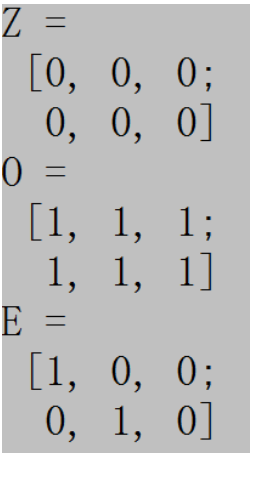展开全文opencv
• 文章目录前言一、创建图像（矩阵）：Mat二、操作图像矩阵的像素 前言 在数字图像处理中，图像的形式实际是一个矩阵，对图像的各种处理也就是对矩阵进行处理。 一、创建图像（矩阵）：Mat 使用Mat创建图像（矩阵）...opencv 计算机视觉 c++
• 转自：https://blog.csdn.net/shenwanjiang111/article/details/54318958Mat 类提供了多种方便的方法来选择图像的局部区域。使用这些方法时需要注意,这些方法并不进行内存的复制操作。如果将局部区域赋值给新的 Mat ...opencv
• QT在跨平台编程中应用越来越广泛，C++中用跨平台开发界面（包括嵌入式设备），QT基本成为第一选择，OpenCV从3.0开始已经慢慢抛弃了过去的C接口，统一改为C++接口，存储图像的IplImage也在被Mat替代，本文主要讲如何...
• 1. Mat数据类型 Mat定义 是一个类，由两个数据部分构成，矩阵头（包含矩阵尺寸，存储方法，存储地址等等）和一个指向存储所有像素值的矩阵的指针 成员变量 int cv::Mat::cols; //返回矩阵的列数 int cv::Mat::...C
• 图片明显错位显示，之后查找了很多资料，终于找到原因，bitmap数据格式中 图片宽度不是4的倍数导致，具体看一下链接 位图文件 https://blog.csdn.net/xiajun07061225/article/details/5813726 位图 最重要的是...
• 最近包装了下C++的人脸识别库以供C#程序调用, 遇到了C#与C库中的图像数据传递的问题...* @Param aWidth: 图像宽度 * @Param aHeight: 图像高度 * @Param aChannel: 图像通道数 灰度图为1, RGB为3 ARGB为4 * @Param a...OpenCv
• //整理创建Mat的方法 //1. 使用Mat()构造函数构建 /** @overload @param rows Number of rows in a 2D array. 二维数组的行数，又图像的高度 ... 二维数组的列数，又图像宽度 @param type Array type. Use ...
• Mat 类提供了多种方便的方法来选择图像的局部区域。使用这些方法时需要注意,这些方法并不进行内存的复制操作。如果将局部区域赋值给新的 Mat 对象,新对象与原始对象共用相同的数据区域,不新申请内存,因此这些方法的...Mat::row
• 创建图像对象的四种方法 Mat 对象的创建有多种方式：克隆、复制、赋值、构造函数。 Mat src = imread("d:/test.jpg"); //读取源地址 构造函数 Mat img_constructe(src); 赋值 Mat img_assign = src; ...opencv python 计算机视觉
• data：Mat对象中的一个指针，指向内存中存放矩阵数据的一块内存 (uchar* data) dims：Mat所代表的矩阵的维度，如 3 * 4 的矩阵为 2 维， 3 * 4 * 5 的为3维 channels：通道，矩阵中的每一个矩阵元素拥有的值的个数，...
• VITIS VISION LIBRARY API REFERENCE xf::Mat Image ... 数据从cv :: Mat显式复制到xf :: Mat，并存储在物理上连续的内存中，以实现最佳性能。 处理后，xf :: Mat中的输出被复制回cv :: Mat以将其写入内存。...
• 格式转换很常见，其实在我实现了 Mat 转 bmp 之后才发现原来 imwrite 接口可以直接将 Mat 数据保存为 .bmp图像，不过下文所谈及的转换是在内存中的转换，因为将图像发送给识别服务器时显然不能先将 Mat 保存为 .bmp ...c++
• 转载出处: ... ...显示RGB图像: [cpp] view plain copy void ShowRGBToWnd(HWND hWnd, BYTE* data, int width, int height)  {
• disimg.cols, //图像宽度 disimg.rows, //图像高度 disimg.data, bitMapinfo, DIB_RGB_COLORS, SRCCOPY); } 获取picture control控件句柄 CWnd *hwnd = GetDlgItem(IDC_STATIC);opencv mfc
• 1. Mat数据类型 Mat定义 是一个类，由两个数据部分构成，矩阵头（包含矩阵尺寸，存储方法，存储地址等等）和一个指向存储所有像素值的矩阵的指针 成员变量 int cv::Mat::cols; //返回矩阵的列数 int cv::Mat::rows ...
• rows 是行数 ， 即图像的高度cols 是列数 ， 即图像宽度这一点要注意，弄反的话会出错当出现错误找不出的时候，不要忘记还有Exception可用try{出错代码}catch（Exception e）{const char *errStr = e.waht();...opencv 错误 异常
• 文章目录图像在内存中的存储方式Mat转Vector类型Vector转Mat 图像在内存中的存储方式 Mat - 基本图像容器：Mat是一个类，由两个数据部分组成：矩阵头（包含矩阵尺寸，存储方法，存储地址等信息）和一个指向存储所有...opencv 计算机视觉 c++
• 一、Mat与Iplimage Mat<-----> Iplimage ：直接赋值 IplImage *iplImg = cvLoadImage("greatwave.jpg", 1); Mat mtx(iplImg); // IplImage* ->Mat 共享数据 // or : Mat mtx = iplImg; cvReleaseImage(&...
• Mat类是OpenCV中基础图像容器类。从根本上来说，一张图像是一个由数值组成的矩阵。这也是OpenCV2用cv::Mat这个数据结构来表示图像的原因。矩阵的每一个元素代表一个像素。对于灰度图像（仅包含“灰色”的图像）而言...OpenCV IplImage 矩阵类
• 在做图像处理中，常用的函数接口有Opencv中的Mat图像类，有时候需要直接用二维指针开辟内存直接存储图像数据，有时候需要用到CxImage类存储图像。本文主要是总结下这三类存储方式之间的图像数据的转换和相应的对应...opencv 新手 c++
• 在数字图像处理中，对一张图片进行傅里叶变换后我们获得的是：实数图像（幅度图像）+虚数图像（相位图像） 傅里叶变换在数字图像处理中将空间域信息转为频域信息。 如果需要得到图像中的几何结构信息，...opencv 卷积 计算机视觉
• Mat类是一个储存矩阵数据的容器，包括灰度、向量、矩阵、彩色图像等数据。Mat类分为矩阵头和指向存储数据的矩阵指针两部分。 矩阵头： 矩阵尺寸： 存储方法: 地址： 引用次数: 而图像传递和复制过程中，主要...opencv 人工智能 自动驾驶
• 目录1 图片格式转换2 png格式转换为Mat3 读写Mat格式的数据 1 图片格式转换 以下代码为Mat、QImage、QPixmap图片格式之间的转换函数： #include <opencv2/opencv.hpp> #include <opencv2/core/core.hpp...opencv c++ 机器视觉...